# Count words and characters in Google Sheets: LEN function

Though word and character count in Google Sheets are used in rare cases, it is still the functionality that some of us expect to see right in the menu. But unlike Google Docs, for Google Sheets, it is the LEN function that does that.

Even though there are many different ways to count characters in spreadsheets, today's blog post will cover the LEN function as its main purpose in tables is to – well, count :) However, it is hardly ever used on its own. Below you will learn how to use Google Sheets LEN correctly and find the most wanted formulas to compute characters in spreadsheets.

## Google Sheets LEN function – usage and syntax

The main and the only purpose of the LEN function in Google Sheets is getting the string length. It is so simple that it even requires only 1 argument:

=LEN(text)
• it can take either the text itself in double-quotes:
`=LEN("Yggdrasil")`
• or a reference to a cell with the text of interest:
`=LEN(A2)`

Let's see if there are any peculiarities in using the function in spreadsheets.

## Character count in Google Sheets

I'll start with the simplest operation: do a character count in Google Sheets the most common way – by referencing a cell with the text using the LEN function.

I enter the formula to B2 and copy it down the entire column to count characters in each row:

`=LEN(A2)`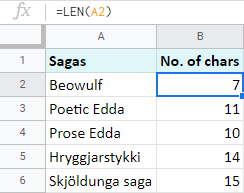Note. The LEN function computes all characters: letters, numbers, spaces, punctuation marks, etc.

You may think that in a similar manner you could do a character count for the entire range of cells, like this: `LEN(A2:A6)`. But, as bizarre as it is, it doesn't simply work this way.

To total characters in several cells, you should wrap your LEN in SUMPRODUCT – the function that tallies up the numbers from entered ranges. In my case, the range is returned by the LEN function:

`=SUMPRODUCT(LEN(A2:A6))`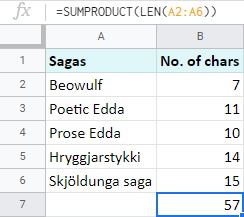Of course, you could incorporate the SUM function instead. But SUM in Google Sheets doesn't process arrays from other functions. To make it work, you will have to add another function – ArrayFormula:

`=ArrayFormula(SUM(LEN(A2:A6)))`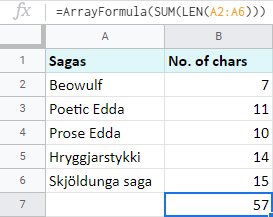## How to count characters without spaces in Google Sheets

As I noted above, Google Sheets LEN function counts each and every character it sees including spaces.

But what if there are extra spaces added by mistake and you don't want to consider them for the result?

For cases like this, there's the TRIM function in Google Sheets. It checks the text for leading, trailing, and repeated spaces in-between. When TRIM is paired with LEN, the latter doesn't count all those odd spaces.

Here's an example. I added spaces in different positions in column A. As you can see, when on its own, Google Sheets LEN counts them all:

`=LEN(A2)`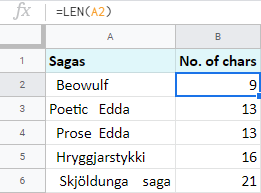But as soon as you integrate TRIM, all extra spaces are ignored:

`=LEN(TRIM(A2))`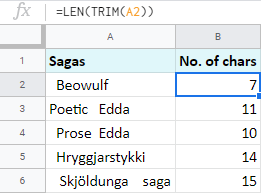You can go further and make your formula disregard even those single spaces between words. The SUBSTITUTE function will assist. Though its main purpose is to replace one character with another, there's a trick to make it reduce spaces completely:

=SUBSTITUTE(text_to_search, search_for, replace_with, [occurrence_number])
• text_to_search is the range you work with: column A, or A2 to be exact.
• search_for should be a space character in double-quotes: " "
• replace_with should contain empty double-quotes. If you're going to ignore spaces, you need to replace them with literally nothing (empty string): ""
• occurence_number is normally used to specify the instance to replace. But since I'm describing how to count characters without all spaces, I suggest you omit this argument as it is optional.

Now try and assemble all these into Google Sheets LEN and you'll see that no space is taken into account:

`=LEN(SUBSTITUTE(A2, " ", ""))`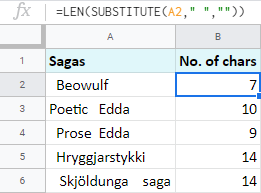## Google Sheets: count specific characters

The same tandem of Google Sheets LEN and SUBSTITUTE is used whenever you need to count specific characters, letters, or numbers.

In my examples, I'm going to find out the number of occurrences for the letter 's'. And this time, I'll start with a ready-made formula:

`=LEN(A2)-LEN(SUBSTITUTE(A2, "s", ""))`

Let's break it down to pieces to understand how it works:

1. SUBSTITUTE(A2, "s", "") looks for the letter 's' in A2 and replaces all occurrences with "nothing", or empty string ("").
2. LEN(SUBSTITUTE(A2, "s", "") works out the number of all characters but 's' in A2.
3. LEN(A2) counts all characters in A2.
4. Finally, you subtract one from the other.

The result difference shows how many 's' there are in the cell: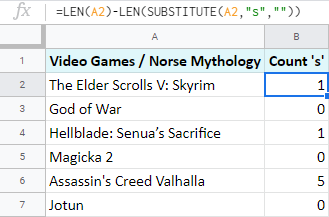Note. You may wonder why B1 says there's only 1 's' in A2 while you can see 3?

The thing is, the SUBSTITUTE function is case-sensitive. I asked it to take all instances of 's' in lowercase and so it did.

To make it ignore text case and process letters in both lower and upper cases, you will have to call one more Google Sheets function for help: LOWER.

It is as simple as Google Sheets LEN and TRIM because all it requires is the text:

`=LOWER(text)`

And all it does is turn the entire text string into lower case. This trick is exactly what you need to make Google Sheets count specific characters no matter their text case:

`=LEN(A2)-LEN(SUBSTITUTE(LOWER(A2), "s", ""))`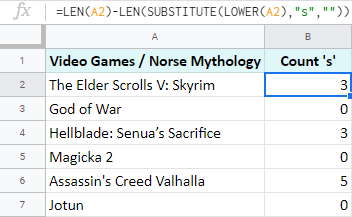Tip. And as before, to count the total of specific characters in the range, wrap your LEN in SUMPRODUCT:

`=SUMPRODUCT(LEN(A2:A7)-LEN(SUBSTITUTE(LOWER(A2:A7), "s", "")))`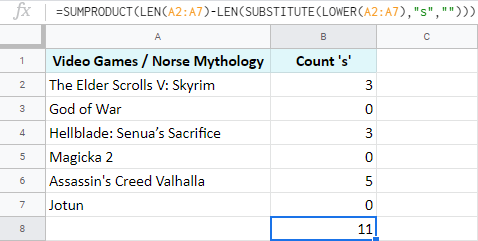## Count words in Google Sheets

When there are multiple words in cells, chances are you will need to have their number instead of the Google Sheets string length.

And though there are multiple ways of doing so, today I will mention how Google Sheets LEN does the job.

Remember the formula I used to count specific characters in Google Sheets? In fact, it will come in handy here as well. Because I'm not going to literally count words. Instead, I will count the number of spaces between the words and then simply add 1. Have a look:

`=LEN(A2)-LEN(SUBSTITUTE((A2), " ", ""))+1`

1. LEN(A2) counts the number of all chars in the cell.
2. LEN(SUBSTITUTE((A2)," ","")) removes all spaces from the text string and counts remaining chars.
3. Then you subtract one from the other, and the difference you get is the number of spaces in the cell.
4. Since words always outnumber spaces in a sentence by one, you add 1 at the end.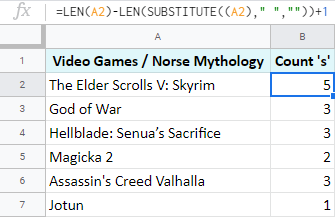## Google Sheets: count specific words

Finally, I'd like to share a Google Sheets formula that you can use to count specific words.

Here I have The Mock Turtle's Song from Alice's Adventures in Wonderland: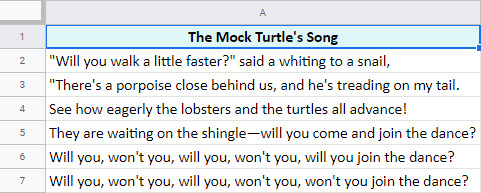I want to know how many times the word 'will' appears in each row. I believe you won't be surprised if I tell you that the formula I need consists of the same functions as before: Google Sheets LEN, SUBSTITUTE, and LOWER:

`=(LEN(A2)-LEN(SUBSTITUTE(LOWER(A2), "will", "")))/LEN("will")`

The formula may look scary but I can assure you that it's easy to comprehend, so bear with me :)

1. Since the text case doesn't matter to me, I use LOWER(A2) to turn everything to lowercase.
2. Then goes SUBSTITUTE(LOWER(A2), "will",""))) – it gets rid of all occurrences of 'will' by replacing them with empty strings ("").
3. After that, I subtract the number of characters without the word 'will' from the total string length. The number I get counts all characters in all occurrences of 'will' in each row.

Thus, if 'will' appears once, the number is 4 as there are 4 letters in the word. If it appears twice, the number is 8, and so on.

4. Finally, I divide this number by the length of the single word 'will'.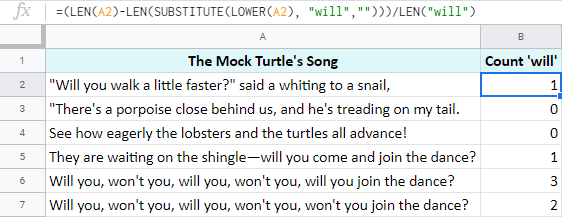Tip. And again, if you'd rather get the total number of all appearances of the word 'will', just enclose the entire formula by SUMPRODUCT:

`=SUMPRODUCT((LEN(A2:A7)-LEN(SUBSTITUTE(LOWER(A2:A7), "will", "")))/LEN("will"))`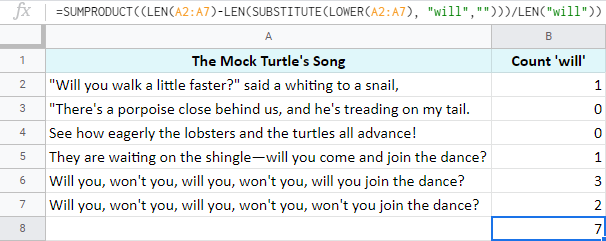As you can see, all these cases of character-count are solved by the same patterns of the same functions for Google Sheets: LEN, SUBSTITUTE, LOWER, and SUMPRODUCT.

If some formulas still confuse you, or if you're not sure how to apply everything to your particular task, don't be shy and ask away in the comments section below!

## You may also be interested inWar in Ukraine! To support Ukraine and save lives please visit this page.